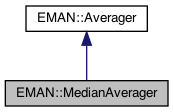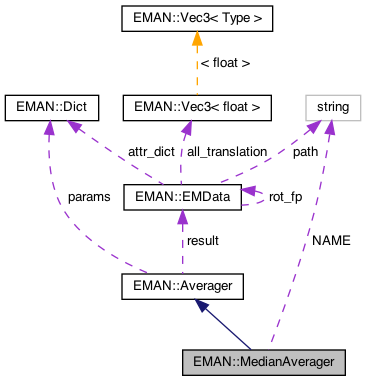EMAN2
EMAN::MedianAverager Class Reference

MedianAverager averages a list of images to the maximum(or minimum of the absolute pixel value) It optionally makes a sigma image. More...

`#include <averager.h>`

Inheritance diagram for EMAN::MedianAverager:[legend]
Collaboration diagram for EMAN::MedianAverager:[legend]

## Public Member Functions

MedianAverager ()

To add an image to the Averager. More...

EMDatafinish ()
Finish up the averaging and return the result. More...

string get_name () const
Get the Averager's name. More...

string get_desc () const

TypeDict get_param_types () const
Get Averager parameter information in a dictionary. More...Public Member Functions inherited from EMAN::Averager
Averager ()

virtual ~Averager ()

virtual void add_image_list (const vector< EMData * > &images)
To add multiple images to the Averager. More...

virtual void set_params (const Dict &new_params)
Set the Averager parameters using a key/value dictionary. More...

virtual void mult (const float &s)
Multiply the result image by some floating point constant This is useful when weighting the input images prior to calling add_image - a situation where it is likely you want to divide by the sum of the weights. More...

## Static Public Member Functions

static AveragerNEW ()

## Static Public Attributes

static const string NAME = "median"

## Private Attributes

std::vector< EMData * > imgsProtected Attributes inherited from EMAN::Averager
Dict params

EMDataresult

## Detailed Description

MedianAverager averages a list of images to the maximum(or minimum of the absolute pixel value) It optionally makes a sigma image.

Parameters
 min If set, will find the min value, otherwise finds max

Definition at line 446 of file averager.h.

## ◆ MedianAverager()

 MedianAverager::MedianAverager ( )

Definition at line 909 of file averager.cpp.

910{
911
912}

Referenced by NEW().

## Member Function Documentation

 void MedianAverager::add_image ( EMData * image )
virtual

To add an image to the Averager.

This image will be averaged in this function.

Parameters
 image The image to be averaged.

Reimplemented from EMAN::Averager.

Definition at line 914 of file averager.cpp.

915{
916 if (!image) {
917 return;
918 }
919
920 if (!imgs.empty() && !EMUtil::is_same_size(image, imgs)) {
921 LOGERR("MedianAverager can only process images of the same size");
922 return;
923 }
924
925 imgs.push_back(image->copy());
926}
static bool is_same_size(const EMData *image1, const EMData *image2)
Check whether two EMData images are of the same size.
Definition: emutil.cpp:1224
std::vector< EMData * > imgs
Definition: averager.h:479
#define LOGERR
Definition: log.h:51

References imgs, EMAN::EMUtil::is_same_size(), and LOGERR.

## ◆ finish()

 EMData * MedianAverager::finish ( )
virtual

Finish up the averaging and return the result.

Returns
The averaged image.

Implements EMAN::Averager.

Definition at line 928 of file averager.cpp.

929{
930 if (imgs.size()==0) return 0;
931 EMData *ret=0;
932
933 if (imgs.size()==1) {
934 ret=imgs;
935 imgs.clear();
936 return ret;
937 }
938
939 // special case for n==2
940 if (imgs.size()==2) {
942 imgs->mult(0.5f);
943 delete imgs;
944 ret=imgs;
945 imgs.clear();
946 return ret;
947 }
948
949 int nx=imgs->get_xsize();
950 int ny=imgs->get_ysize();
951 int nz=imgs->get_zsize();
952
953 // special case for n==3
954 if (imgs.size()==3) {
955 for (int z=0; z<nz; z++) {
956 for (int y=0; y<ny; y++) {
957 for (int x=0; x<nx; x++) {
958 float v0=imgs->get_value_at(x,y,z);
959 float v1=imgs->get_value_at(x,y,z);
960 float v2=imgs->get_value_at(x,y,z);
961
962 if (v0<=v1) {
963 if (v0>=v2) continue;
964 if (v1<=v2) imgs->set_value_at(x,y,z,v1);
965 else imgs->set_value_at(x,y,z,v2);
966 }
967 else {
968 if (v0<=v2) continue;
969 if (v2<=v1) imgs->set_value_at(x,y,z,v1);
970 else imgs->set_value_at(x,y,z,v2);
971 }
972 }
973 }
974 }
975
976 delete imgs;
977 delete imgs;
978 ret=imgs;
979 imgs.clear();
980 return ret;
981 }
982
983 ret=imgs->copy();
984 std::vector<float> vals(imgs.size(),0.0f);
985
986
987 for (int z=0; z<nz; z++) {
988 for (int y=0; y<ny; y++) {
989 for (int x=0; x<nx; x++) {
990 int i=0;
991 for (std::vector<EMData *>::iterator it = imgs.begin() ; it != imgs.end(); ++it,++i) {
992 vals[i]=(*it)->get_value_at(x,y,z);
993 }
994
995 std::sort(vals.begin(),vals.end());
996 //printf("%d %d %d %d\n",x,y,z,vals.size());
997 if (vals.size()&1) ret->set_value_at(x,y,z,vals[vals.size()/2]); // for even sizes, not quite right, and should include possibility of local average
998 else ret->set_value_at(x,y,z,(vals[vals.size()/2]+vals[vals.size()/2-1])/2.0f);
999 }
1000 }
1001 }
1002
1003 if (!imgs.empty()) {
1004 for (std::vector<EMData *>::iterator it = imgs.begin() ; it != imgs.end(); ++it) if (*it) delete *it;
1005 imgs.clear();
1006 }
1007
1008
1009 return ret;
1010}
#define v0(i)
Definition: analyzer.cpp:698
EMData stores an image's data and defines core image processing routines.
Definition: emdata.h:82
#define y(i, j)
Definition: projector.cpp:1516
#define x(i)
Definition: projector.cpp:1517

References imgs, v0, x, and y.

## ◆ get_desc()

 string EMAN::MedianAverager::get_desc ( ) const
inlinevirtual

Implements EMAN::Averager.

Definition at line 459 of file averager.h.

460 {
461 return "Computes the median value instead of the mean. If even number of images, averages the middle two.";
462 }

## ◆ get_name()

 string EMAN::MedianAverager::get_name ( ) const
inlinevirtual

Get the Averager's name.

Each Averager is identified by a unique name.

Returns
The Averager's name.

Implements EMAN::Averager.

Definition at line 454 of file averager.h.

455 {
456 return NAME;
457 }
static const string NAME
Definition: averager.h:476

References NAME.

## ◆ get_param_types()

 TypeDict EMAN::MedianAverager::get_param_types ( ) const
inlinevirtual

Get Averager parameter information in a dictionary.

Each parameter has one record in the dictionary. Each record contains its name, data-type, and description.

Returns
A dictionary containing the parameter info.

Reimplemented from EMAN::Averager.

Definition at line 469 of file averager.h.

470 {
471 TypeDict d;
472 //d.put("min", EMObject::INT, "If set, will average to minimum absolute value, by default average to max");
473 return d;
474 }

## ◆ NEW()

 static Averager * EMAN::MedianAverager::NEW ( )
inlinestatic

Definition at line 464 of file averager.h.

465 {
466 return new MedianAverager();
467 }

References MedianAverager().

## ◆ imgs

 std::vector EMAN::MedianAverager::imgs
private

Definition at line 479 of file averager.h.